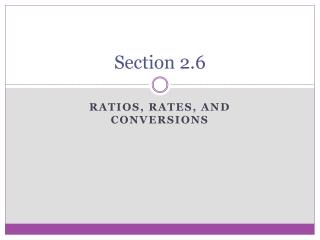DownloadDownload PresentationSection 2.6

# Section 2.6

Download Presentation## Section 2.6

- - - - - - - - - - - - - - - - - - - - - - - - - - - E N D - - - - - - - - - - - - - - - - - - - - - - - - - - -
##### Presentation Transcript

1. Section 2.6 Ratios, Rates, and conversions

2. Ratio • Compares two numbers by division • Can be written in 3 ways: a a : b a to b b Must always be simplified!! • Meaning – for every a units of one quantity, you have b units of another quantity • Example: the number of boys to girls in class is 2 to 1

3. Rate • A ratio that compares quantities measured in different units • A rate with a denominator of 1 unit is called a unit rate

4. Comparing Unit Rates • Write each ratio as a unit rate THEN compare • Example: • You are shopping for T-shirts. Which store offers the better deal? • Store A: \$25 for 2 shirts • Store B: \$45 for 4 shirts • Store C: \$30 for 3 shirts

5. Converting Units • To convert from one unit to another, such as feet to inches, you multiply the original unit by a conversion factor that produces the desired unit. • Conversion factor – a ratio of two equivalent measures in different units • Conversion factors ALWAYS equal to 1 • ALWAYS place the given conversion on the bottom on the conversion factor • Example: 1 ft 12 in **** PAGE 723 has Conversions

6. Example • Convert the given amount to the given unit • 330 min; hours ● 15 kg; grams • 330 min · 1 h 60 min ● 5 ft 3in; inches

7. Converting Rates with 2 Different Units • You must multiply by two conversion factors in order to change both of the units. • Example: • A student ran the 50-yard dash in 5.8 seconds. At what speed did the student run in miles per hour? Round your answer to the nearest tenth. 50 yards 5.8 sec